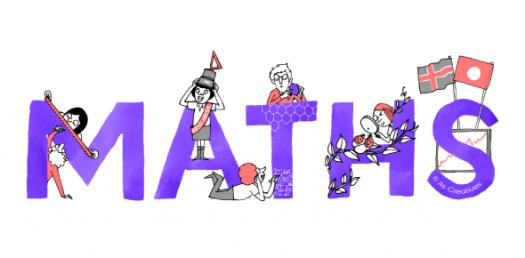# 6th Grade Maths Quiz: Can You Pass?

8 Questions | Total Attempts: 31065SettingsYou may have gone to college, but do you think you are well capable of handling some math problems that 6th graders face daily? If you believe in yourself so much, then take up the quiz below and get to prove yourself. Remember to choose the right answer and share your scores, also share this quiz with your siblings studying in 6th grade. All the best of luck!

Related Topics
• 1.
What value of "x" makes the equation below true? -15 + (-5x) = 0
• A.

5

• B.

3

• C.

-3

• D.

-5

• 2.
Two angles of a triangle measure 35 degrees and 65 degrees. What is the measure of the third angle of the triangle?
• A.

100 degrees

• B.

80 degrees

• C.

125 degrees

• D.

135 degrees

• 3.
• A.

180 degrees

• B.

270 degrees

• C.

360 degrees

• D.

120 degrees

• 4.
Ratio of 500ml to 2 litres is:
• A.

1:4

• B.

1:2

• C.

1:8

• D.

1:5

• 5.
Which of the following is true?
• A.

20 + 0 = 0

• B.

0 * 20 = 0

• C.

20 - 0 = 0

• D.

20 - 20 - 0 = 20

• 6.
• A.

0

• B.

1

• C.

9

• D.

10

• 7.
• A.

3

• B.

3/10

• C.

3/100

• D.

3/1000

• 8.
Which of these numbers have only two factors, 1 and the number itself?
• A.

37+1

• B.

37+37

• C.

37*37

• D.

37*1